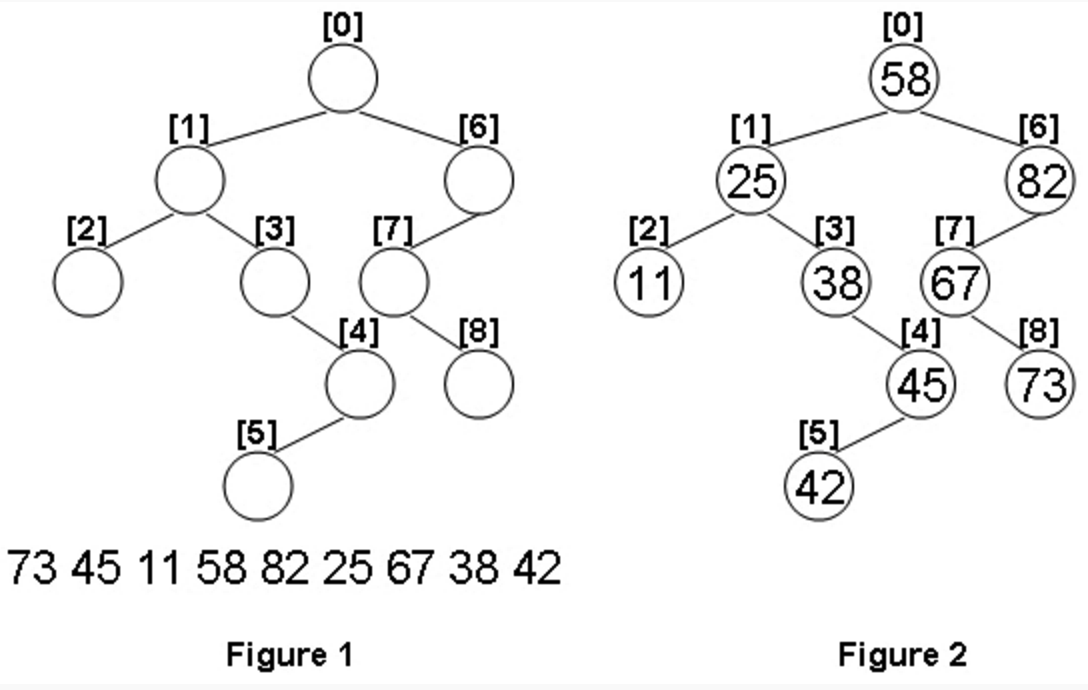# 1099. Build A Binary Search Tree (30)-PAT甲级真题

A Binary Search Tree (BST) is recursively defined as a binary tree which has the following properties:

The left subtree of a node contains only nodes with keys less than the node’s key.
The right subtree of a node contains only nodes with keys greater than or equal to the node’s key.
Both the left and right subtrees must also be binary search trees.
Given the structure of a binary tree and a sequence of distinct integer keys, there is only one way to fill these keys into the tree so that the resulting tree satisfies the definition of a BST. You are supposed to output the level order traversal sequence of that tree. The sample is illustrated by Figure 1 and 2.Input Specification:

Each input file contains one test case. For each case, the first line gives a positive integer N (<=100) which is the total number of nodes in the tree. The next N lines each contains the left and the right children of a node in the format “left_index right_index”, provided that the nodes are numbered from 0 to N-1, and 0 is always the root. If one child is missing, then -1 will represent the NULL child pointer. Finally N distinct integer keys are given in the last line.

Output Specification:

For each test case, print in one line the level order traversal sequence of that tree. All the numbers must be separated by a space, with no extra space at the end of the line.

Sample Input:
9
1 6
2 3
-1 -1
-1 4
5 -1
-1 -1
7 -1
-1 8
-1 -1
73 45 11 58 82 25 67 38 42
Sample Output:
58 25 82 11 38 67 45 73 42

2. 因为是二叉搜索树，所以中序遍历这棵树得到的结点顺序应该是给出的数值序列从小到大的排列顺序，所以把数值序列排序后，可以在中序遍历的时候直接赋值当前a[root].data～同时可得知树的最大层数maxLevel的值～
3. 二维数组v用来存储每一层的结点下标，一共有0到maxLevel层。用for循环从0开始一层层遍历v，就可以得到下一层的l和r，遍历过程中可以输出每个结点对应的data值a[v[i][j]].data～